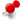# How to modify the values of existing equation plot in plot plugin?ClassicListThreaded3 messagesOpen this post in threaded view
|

## How to modify the values of existing equation plot in plot plugin?

 Hi, Let's say I've plotted a curve by specifying some equation in the plot plugin. For ex, plugins>plot plugin. But how do I access the equation of that existing curve again to modify some parameters? Thanks.